# Groebner Basis for Linear Network Coding in Sage

2018-02-08, updated 2020-06-21 —   ⇦Transfer Functions in pythonMulti-audio (In development)⇨

Backup of ./html/Groebner_Basis.html Here is the pdf with better formatting: GroebnerBasis–Sage

This tests the solvability of a linear network code by seeing if the linear network coding ideal is a non-trivial ideal, which implies that there is a solution to it.

# Usage

Install sage and libsingular.

get_groebner_basis(field, graph, sources, sinks)

# Limitations

Does not support multi-edges

## Inputs

Field is a type of field. Example:

GF(2)
The type of graph is a labelled digraph. The nodes need to be given names in order for the sinks and sources to be mapped. Example:

DiGraph({'s': ['t','u'], 't': ['x','y'], 'u': ['x','z'], 'x': ['y','z']})
The type of sources is a dictionary of lists, mapping a source node name to the list of processes inside it. Example:

sources = {'s': [0,1]}
The type of sinks is a dictionary of dictionary of lists, mapping a sink node name to to its demands. The demands are a dictionary mapping the sources from which the sink demands, to the processes from that source that are demanded. Example:

sinks = {'y': {'s':[0,1]} , 'z': {'s':[0,1]}}

## Returns

It returns the groebner basis for that particular multicast network

-------------------------------------------------------------------------------------------

Generates the sink matrix B to calculate the transfer matrix, defined as $\left(A\left(1-F{\right)}^{-1}{B}^{T}\right)$$(A (1-F)^{-1} B^T)$

def generate_matrix_sink(sinks, graph): vertices = graph.vertices() m = [] for s in sinks: sources = sinks[s] for source in sources: sink_source_rows = sources[source] for i in sink_source_rows: row = [] for v in vertices: origin, destination, edge_name = v if destination == s: row.append(var('sink_'+str(origin)+str(destination)+str(source) + str(i))) else: row.append(0) m.append(row) return matrix(SR, m, sparse=True)

Generates the source matrix A to calculate the transfer matrix, defined as $\left(A\left(1-F{\right)}^{-1}{B}^{T}\right)$$(A (1-F)^{-1} B^T)$

def generate_matrix_source(sources, graph): vertices = graph.vertices() m = [] for s in sources: source_rows = sources[s] for i in source_rows: row = [] for v in vertices: origin, destination, edge_name = v if origin == s: row.append(var('source_'+str(origin)+str(destination)+str(i))) else: row.append(0) m.append(row) return matrix(SR, m, sparse=True)

Generates the source matrix F to calculate the transfer matrix, defined as $\left(A\left(1-F{\right)}^{-1}{B}^{T}\right)$$(A (1-F)^{-1} B^T)$

def generate_labeled_line_graph_matrix(graph): line_graph = graph.line_graph() vertices = line_graph.vertices() adj_matrix = line_graph.adjacency_matrix() n_rows = adj_matrix.nrows() n_cols = adj_matrix.ncols() m = [[y for y in x] for x in adj_matrix] for i in range(n_rows): for j in range(n_cols): origin_1, destination_1, edge_name_1 = vertices[i] origin_2, destination_2, edge_name_2 = vertices[j] m[i][j] = m[i][j] * var('e_'+str(origin_1)+str(destination_1)+'_' +str(origin_2)+str(destination_2)) return matrix(SR, m, sparse=True)

Genenerates the transfer matrix $\left(A\left(1-F{\right)}^{-1}{B}^{T}\right)$$(A (1-F)^{-1} B^T)$, that maps inputs to outputs

def generate_transfer_matrix(graph, sources, sinks): line_graph = graph.line_graph() F = generate_labeled_line_graph_matrix(graph) I = matrix.identity(F.nrows()) A = generate_matrix_source(sources, line_graph) B = generate_matrix_sink(sinks, line_graph) return A*~(I-F)*B.T

Helper functions to find which rows in A and B are assigned to which source process or to which sink.

def get_source_process_rows(source_process_list, sources): source_rows ={} i=0 for s in sources: source_rows[s] = {} dic = source_rows[s] for process in sources[s]: dic[process] = i i+=1 rows = [source_rows[source][process] for source in source_process_list for process in source_process_list[source]] return rows def get_sink_rows(sink_name, sinks): sink_rows ={} i=0 for s in sinks: sink = sinks[s] len_sink = sum([len(sink[source]) for source in sink]) sink_rows[s] = [] for j in range(len_sink): sink_rows[s].append(i) i+=1 rows = sink_rows[sink_name] return rows

Given the sink demands and the sources, these helper functions find which submatrices of the transfer function need to have determinant different from 0 and which entries need to be equal to 0.

def generate_nonnull_matrices(sources, sinks): nonnull_matrices = [] for si in sinks: rows = get_source_process_rows(sinks[si], sources) columns = get_sink_rows(si, sinks) nonnull_matrices.append((rows, columns)) return nonnull_matrices def generate_null_elements(sources, sinks): n_processes = sum([len(sources[s]) for s in sources]) n_demands = sum([len(sinks[sink][source]) for sink in sinks for source in sinks[sink]]) m = [[1 for i in range(n_demands)] for j in range(n_processes)] nonnull_matrices = generate_nonnull_matrices(sources, sinks) for rows,columns in nonnull_matrices: for r in rows: for c in columns: m[r][c] = 0 return m

Calculates the ideal of the linear network problem, as stated on http://www.mit.edu/~medard/mpapers/aaatnetworkcoding.pdf

It is the ideal of all values that should be equal to 0.

def get_ideal(graph, sources, sinks): transfer_matrix = generate_transfer_matrix(graph, sources, sinks) nonnull_matrices = generate_nonnull_matrices(sources, sinks) null_elements_indexes = generate_null_elements(sources, sinks) null_elements = [transfer_matrix[i][j] for i in range(len(null_elements_indexes)) for j in range(len(null_elements_indexes)) if null_elements_indexes[i][j]==1] nonnull_prod = reduce((lambda x,y: x*y), (transfer_matrix.matrix_from_rows_and_columns(*row_column).det() for row_column in nonnull_matrices)) null_ideal = [1- var('normalizer')*nonnull_prod] + null_elements return null_ideal

Calculates the groebner basis of the ideal of the linear network problem.

def get_groebner_basis(field, graph, sources, sinks): ideal = get_ideal(graph, sources, sinks) variables = [v for x in ideal for v in x.variables()] R = PolynomialRing(field, [x for x in set(variables)]) I = R*ideal basis = I.groebner_basis() return basis

# Example

%time butterflyGraph = DiGraph({'s': ['t','u'], 't': ['x','y'], 'u': ['x','z'], 'x': ['y','z']}) butterflyGraph.plot().show() butterflysources = {'s': [0,1]} butterflysinks = {'y': {'s':[0,1]} , 'z': {'s':[0,1]}} g = get_groebner_basis(GF(2), butterflyGraph, butterflysources, butterflysinks) g2 = get_groebner_basis(GF(3), butterflyGraph, butterflysources, butterflysinks) print "has solution in GF2 => " + str(g != ) + "\n groebner_basis = " + str(g) print "" print "has solution in GF3 => " + str(g2 != ) + "\n groebner_basis = " + str(g2) print "6 nodes, 8 edges, 1 sources, 2 sinks"
 has solution in GF2 => True groebner_basis = [e_ux_xy*e_tx_xz*source_su0^2*e_st_tx*sink_uzs0*source_st1^2*sink_ty\ s0*e_su_uz*e_st_ty*sink_xzs1*sink_xys1*normalizer*e_su_ux + e_ux_xy*e_tx_xz*e_st_tx*source_su1^2*sink_uzs0*sink_tys0*e_su_uz*sou\ rce_st0^2*e_st_ty*sink_xzs1*sink_xys1*normalizer*e_su_ux + e_ux_xy*e_tx_xz*source_su0^2*e_st_tx*source_st1^2*sink_tys0*e_su_uz*\ e_st_ty*sink_xys1*normalizer*sink_xzs0*sink_uzs1*e_su_ux + e_ux_xy*e_tx_xz*e_st_tx*source_su1^2*sink_tys0*e_su_uz*source_st0^2*\ e_st_ty*sink_xys1*normalizer*sink_xzs0*sink_uzs1*e_su_ux + e_ux_xy*e_tx_xz*source_su0^2*e_st_tx*sink_tys1*sink_uzs0*source_st1^\ 2*e_su_uz*e_st_ty*sink_xzs1*normalizer*e_su_ux*sink_xys0 + e_ux_xy*e_tx_xz*e_st_tx*source_su1^2*sink_tys1*sink_uzs0*e_su_uz*sou\ rce_st0^2*e_st_ty*sink_xzs1*normalizer*e_su_ux*sink_xys0 + e_ux_xy*e_tx_xz*source_su0^2*e_st_tx*sink_tys1*source_st1^2*e_su_uz*\ e_st_ty*normalizer*sink_xzs0*sink_uzs1*e_su_ux*sink_xys0 + e_ux_xy*e_tx_xz*e_st_tx*source_su1^2*sink_tys1*e_su_uz*source_st0^2*\ e_st_ty*normalizer*sink_xzs0*sink_uzs1*e_su_ux*sink_xys0 + 1] has solution in GF3 => True groebner_basis = [e_ux_xy*e_tx_xz*source_su0^2*e_st_tx*sink_uzs0*source_st1^2*sink_ty\ s0*e_su_uz*e_st_ty*sink_xzs1*sink_xys1*normalizer*e_su_ux + e_ux_xy*e_tx_xz*source_su0*e_st_tx*source_su1*sink_uzs0*source_st1*s\ ink_tys0*e_su_uz*source_st0*e_st_ty*sink_xzs1*sink_xys1*normalizer*e\ _su_ux + e_ux_xy*e_tx_xz*e_st_tx*source_su1^2*sink_uzs0*sink_tys0*e_su_uz*sou\ rce_st0^2*e_st_ty*sink_xzs1*sink_xys1*normalizer*e_su_ux - e_ux_xy*e_tx_xz*source_su0^2*e_st_tx*source_st1^2*sink_tys0*e_su_uz*\ e_st_ty*sink_xys1*normalizer*sink_xzs0*sink_uzs1*e_su_ux - e_ux_xy*e_tx_xz*source_su0*e_st_tx*source_su1*source_st1*sink_tys0*e\ _su_uz*source_st0*e_st_ty*sink_xys1*normalizer*sink_xzs0*sink_uzs1*e\ _su_ux - e_ux_xy*e_tx_xz*e_st_tx*source_su1^2*sink_tys0*e_su_uz*source_st0^2*\ e_st_ty*sink_xys1*normalizer*sink_xzs0*sink_uzs1*e_su_ux - e_ux_xy*e_tx_xz*source_su0^2*e_st_tx*sink_tys1*sink_uzs0*source_st1^\ 2*e_su_uz*e_st_ty*sink_xzs1*normalizer*e_su_ux*sink_xys0 - e_ux_xy*e_tx_xz*source_su0*e_st_tx*source_su1*sink_tys1*sink_uzs0*so\ urce_st1*e_su_uz*source_st0*e_st_ty*sink_xzs1*normalizer*e_su_ux*sin\ k_xys0 - e_ux_xy*e_tx_xz*e_st_tx*source_su1^2*sink_tys1*sink_uzs0*e_su_uz*sou\ rce_st0^2*e_st_ty*sink_xzs1*normalizer*e_su_ux*sink_xys0 + e_ux_xy*e_tx_xz*source_su0^2*e_st_tx*sink_tys1*source_st1^2*e_su_uz*\ e_st_ty*normalizer*sink_xzs0*sink_uzs1*e_su_ux*sink_xys0 + e_ux_xy*e_tx_xz*source_su0*e_st_tx*source_su1*sink_tys1*source_st1*e\ _su_uz*source_st0*e_st_ty*normalizer*sink_xzs0*sink_uzs1*e_su_ux*sin\ k_xys0 + e_ux_xy*e_tx_xz*e_st_tx*source_su1^2*sink_tys1*e_su_uz*source_st0^2*\ e_st_ty*normalizer*sink_xzs0*sink_uzs1*e_su_ux*sink_xys0 + 1] 6 nodes, 8 edges, 1 sources, 2 sinks CPU time: 0.23 s, Wall time: 0.23 s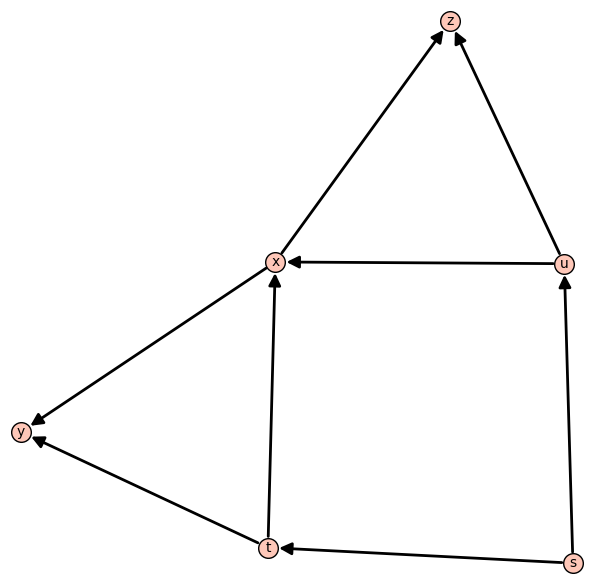M network, from

On Coding for Non-Multicast Networks, by Medard, Muriel and Effros, Michelle and Karger, David and Ho

This network has no scalar solution
%time mnetworkGraph = DiGraph({1: [3,4], 2: [4,5], 3: [6,7,8,9], 4: [6,7,8,9], 5: [6,7,8,9]}) mnetworkGraph.plot().show() mnetworksources = {1: ['a','A'], 2:['b','B']} mnetworksinks = {6: {1:['a'], 2:['b']}, 7: {1:['a'], 2:['B']}, 8: {1:['A'], 2:['b']}, 9: {1:['A'], 2:['B']}} g = get_groebner_basis(GF(2), mnetworkGraph, mnetworksources, mnetworksinks) g2 = get_groebner_basis(GF(3), mnetworkGraph, mnetworksources, mnetworksinks) print "has solution in GF2 => " + str(g != ) + "\n groebner_basis = " + str(g) print "" print "has solution in GF3 => " + str(g2 != ) + "\n groebner_basis = " + str(g2) print "9 nodes, 16 edges, 2 sources, 4 sinks"
 has solution in GF2 => False groebner_basis =  has solution in GF3 => False groebner_basis =  9 nodes, 16 edges, 2 sources, 4 sinks CPU time: 0.56 s, Wall time: 0.56 s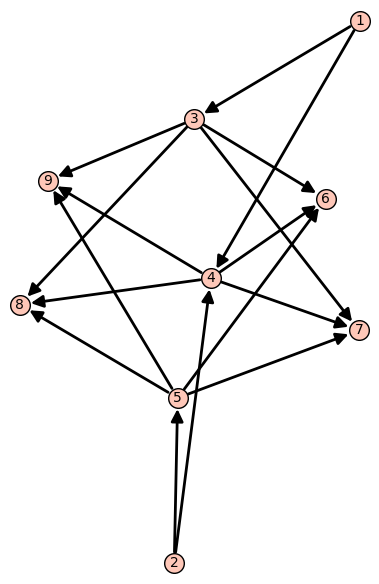It has solution over even fields but no solution over odd fields.

%time network1Graph = DiGraph({4: [13,37], 5: [13,14], 6: [14,22], 13: , 14: , 17: [21, 22], 18: [21, 39], 21: , 22: , 29: [37, 38], 30: [38, 39]}) network1Graph.plot().show() network1sources = {4: ['a'], 5:['b'], 6:['c']} network1sinks = {39: {4:['a']}, 38: {5:['b']}, 37: {6:['c']}} g = get_groebner_basis(GF(2), network1Graph, network1sources, network1sinks) g2 = get_groebner_basis(GF(3), network1Graph, network1sources, network1sinks) print "has solution in GF2 => " + str(g != ) + "\n groebner_basis = " + str(g) print "" print "has solution in GF3 => " + str(g2 != ) + "\n groebner_basis = " + str(g2) print "14 nodes, 18 edges, 3 sources, 3 sinks"
 has solution in GF2 => True groebner_basis = Polynomial Sequence with 25 Polynomials in 29 Variables has solution in GF3 => False groebner_basis =  14 nodes, 18 edges, 3 sources, 3 sinks CPU time: 0.53 s, Wall time: 0.53 s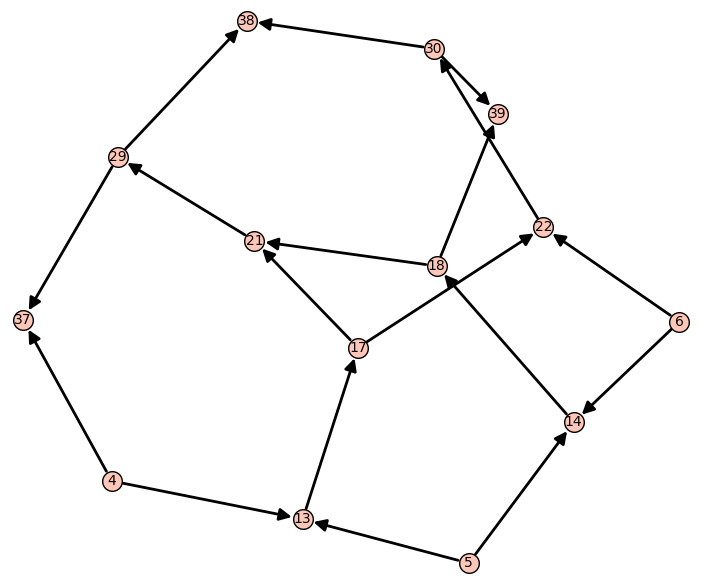Network N2 from http://ieeexplore.ieee.org/stamp/stamp.jsp?tp=&arnumber=1468297

It has a scalar linear solution over any ring where is a unit, but has no linear solution for any vector dimension over a finite field with characteristic two. Also, the coding capacity of is .

%time network2Graph = DiGraph({3: [9,10], 7: [23,15,24], 8: [23,15,25], 9: [15, 24, 25], 10: [26, 16, 27], 11: [26, 16, 28], 12: [16, 27, 28], 15: , 16: , 19: [40, 41, 42], 20: [44, 45, 46], 23: , 24: , 25: , 26: , 27: , 28: , 31: [40,43], 32: [41,43], 33: [42,43], 34: [44,43], 35: [45,43], 36: [46,43]}) network2Graph.plot().show() network2sources = {3: ['c'], 7:['a'], 8:['b'], 11:['d'], 12:['e']} network2sinks = {40: {3:['c']}, 41: {8:['b']}, 42: {7:['a']}, 43: {3:['c']}, 44: {12:['e']}, 45: {11:['d']}, 46: {3:['c']}} g = get_groebner_basis(GF(2), network2Graph, network2sources, network2sinks) g2 = get_groebner_basis(GF(3), network2Graph, network2sources, network2sinks) print "has solution in GF2 => " + str(g != ) + "\n groebner_basis = " + str(g) print "" print "has solution in GF3 => " + str(g2 != ) + "\n groebner_basis = " + str(g2) print "30 nodes, 46 edges, 5 sources, 7 sinks"
 has solution in GF3 => True groebner_basis = Polynomial Sequence with 2025 Polynomials in 75 Variables 30 nodes, 46 edges, 5 sources, 7 sinks CPU time: 4.58 s, Wall time: 4.60 s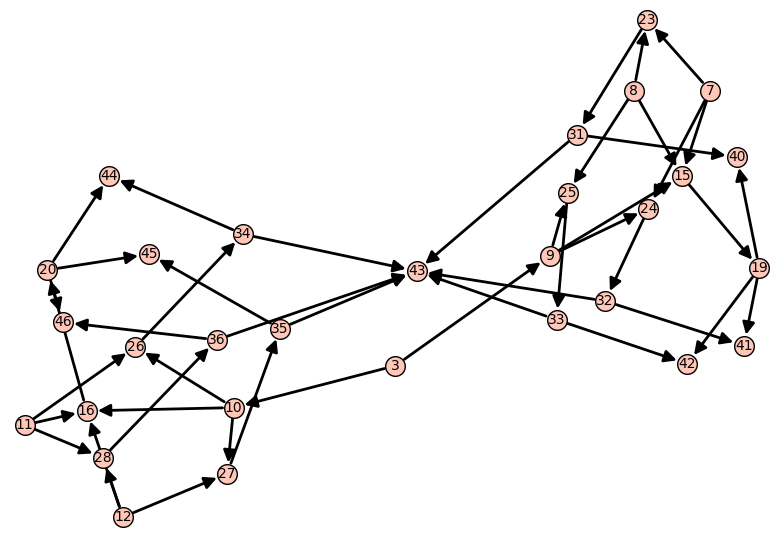Network N3 from http://ieeexplore.ieee.org/stamp/stamp.jsp?tp=&arnumber=1468297

It has no scalar nor vector solutions.

%time network3Graph = DiGraph({4: [13,37], 5: [13,14], 6: [14,22], 13: , 14: , 17: [21, 22], 18: [21, 39], 21: , 22: , 29: [37, 38], 30: [38, 39], 3: [6,9,10], 7: [23,15,24], 8: [23,15,25], 9: [15, 24, 25], 10: [26, 16, 27], 11: [26, 16, 28], 12: [16, 27, 28], 15: , 16: , 19: [40, 41, 42], 20: [44, 45, 46], 23: , 24: , 25: , 26: , 27: , 28: , 31: [40,43], 32: [41,43], 33: [42,43], 34: [44,43], 35: [45,43], 36: [46,43], 1: [4,7], 2: [5,8]}) network3Graph.plot().show() network3sources = {1: ['a'], 2:['b'], 3: ['c'], 11:['d'], 12:['e']} network3sinks = {40: {3:['c']}, 41: {2:['b']}, 42: {1:['a']}, 43: {3:['c']}, 44: {12:['e']}, 45: {11:['d']}, 46: {3:['c']}, 39: {1:['a']}, 38: {2:['b']}, 37: {3:['c']}} g = get_groebner_basis(GF(2), network3Graph, network3sources, network3sinks) g2 = get_groebner_basis(GF(3), network3Graph, network3sources, network3sinks) print "has solution in GF2 => " + str(g != ) + "\n groebner_basis = " + str(g) print "" print "has solution in GF3 => " + str(g2 != ) + "\n groebner_basis = " + str(g2) print "46 nodes, 69 edges, 5 sources, 10 sinks"
 has solution in GF2 => False groebner_basis =  has solution in GF3 => False groebner_basis =  46 nodes, 69 edges, 5 sources, 10 sinks CPU time: 2.52 s, Wall time: 2.52 s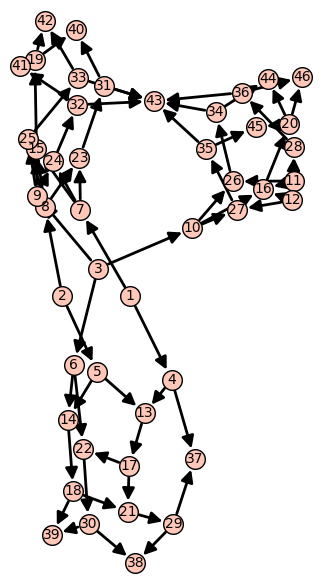Network N1 From:

Unachievability of Network Coding Capacity
Randall Dougherty, Chris Freiling, and Kenneth Zeger, Fellow, IEEE

Only has solution over alphabets that have property P, as defined in the paper

%time networkN1Graph = DiGraph({1: [4,6,7], 2: [6,4,8], 3: [4,7,8], 4: , 5: [12,13,14], 6: , 7: , 8: , 9: , 10: , 11: }) networkN1Graph.plot().show() networkN1Sources = {1: ['a'], 2:['b'], 3:['c']} networkN1Sinks = {12: {3:['c']}, 13: {2:['b']}, 14: {1:['a']}} g = get_groebner_basis(GF(2), networkN1Graph, networkN1Sources, networkN1Sinks) g2 = get_groebner_basis(GF(3), networkN1Graph, networkN1Sources, networkN1Sinks) print "has solution in GF2 => " + str(g != ) + "\n groebner_basis = " + str(g) print "" print "has solution in GF3 => " + str(g2 != ) + "\n groebner_basis = " + str(g2) print str(networkN1Graph.order()) + " nodes, " + str(networkN1Graph.size()) +" edges, 3 sources, 3 sinks"
 has solution in GF2 => True groebner_basis = Polynomial Sequence with 22 Polynomials in 31 Variables has solution in GF3 => True groebner_basis = Polynomial Sequence with 22 Polynomials in 31 Variables 14 nodes, 19 edges, 3 sources, 4 sinks CPU time: 0.38 s, Wall time: 0.39 s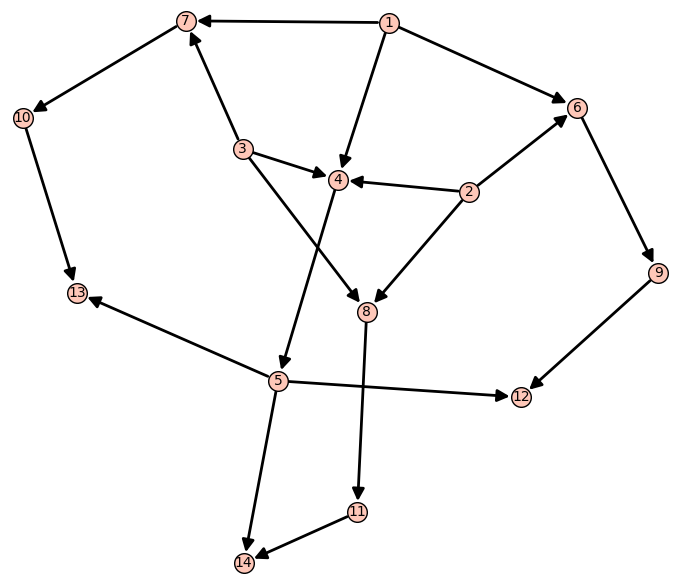Network N2 From:

Unachievability of Network Coding Capacity
Randall Dougherty, Chris Freiling, and Kenneth Zeger, Fellow, IEEE

Only has solution over alphabets that have property P, as defined in the paper, and no elements of order 2.

%time networkN2Graph = DiGraph({1: [4,6,7], 2: [6,4,8], 3: [4,7,8], 4: , 5: [12,13,14], 6: , 7: , 8: , 9: [12, 15], 10: [13, 15], 11: [14, 15]}) networkN2Graph.plot().show() networkN2Sources = {1: ['a'], 2:['b'], 3:['c']} networkN2Sinks = {12: {3:['c']}, 13: {2:['b']}, 14: {1:['a']}, 15: {3:['c']}} g = get_groebner_basis(GF(2), networkN2Graph, networkN2Sources, networkN2Sinks) g2 = get_groebner_basis(GF(3), networkN2Graph, networkN2Sources, networkN2Sinks) print "has solution in GF2 => " + str(g != ) + "\n groebner_basis = " + str(g) print "" print "has solution in GF3 => " + str(g2 != ) + "\n groebner_basis = " + str(g2) print str(networkN2Graph.order()) + " nodes, " + str(networkN2Graph.size()) +" edges, 3 sources, 4 sinks"
 has solution in GF2 => False groebner_basis =  has solution in GF3 => True groebner_basis = Polynomial Sequence with 57 Polynomials in 37 Variables 15 nodes, 22 edges, 3 sources, 4 sinks CPU time: 0.47 s, Wall time: 0.47 s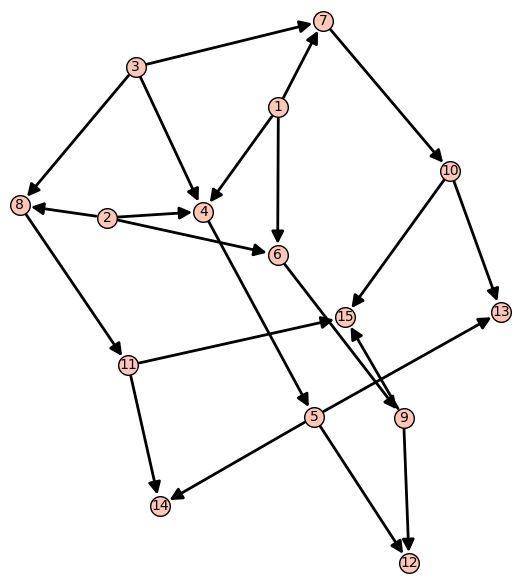Network N3 From:

Unachievability of Network Coding Capacity
Randall Dougherty, Chris Freiling, and Kenneth Zeger, Fellow, IEEE

Only has solution over alphabets that have property P, as defined in the paper, and all non zero elements have order 2.

%time networkN3Graph = DiGraph({16: [19, 27], 17: [19, 20], 18: [20, 24], 19: , 20: , 21: [23, 24], 22: [23, 29], 23: , 24: , 25: [27, 28], 26: [28, 29]}) networkN3Graph.plot().show() networkN3Sources = {16: ['a'], 17:['b'], 18:['c']} networkN3Sinks = {27: {18:['c']}, 28: {17:['b']}, 29: {16:['a']} } g = get_groebner_basis(GF(2), networkN3Graph, networkN3Sources, networkN3Sinks) g2 = get_groebner_basis(GF(3), networkN3Graph, networkN3Sources, networkN3Sinks) g3 = get_groebner_basis(GF(4), networkN3Graph, networkN3Sources, networkN3Sinks) print "has solution in GF2 => " + str(g != ) + "\n groebner_basis = " + str(g) print "" print "has solution in GF3 => " + str(g2 != ) + "\n groebner_basis = " + str(g2) print "" print "has solution in GF4 => " + str(g3 != ) + "\n groebner_basis = " + str(g3) print str(networkN3Graph.order()) + " nodes, " + str(networkN3Graph.size()) +" edges, 3 sources, 3 sinks"
 has solution in GF2 => True groebner_basis = Polynomial Sequence with 25 Polynomials in 29 Variables has solution in GF3 => False groebner_basis =  has solution in GF4 => True groebner_basis = Polynomial Sequence with 25 Polynomials in 29 Variables 14 nodes, 18 edges, 3 sources, 3 sinks CPU time: 0.57 s, Wall time: 0.60 s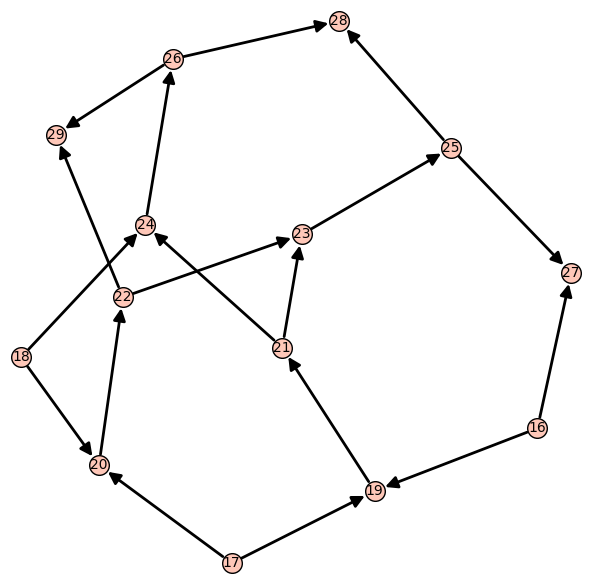## 2 CSS

Date: 2020-09-10 Thu 21:04